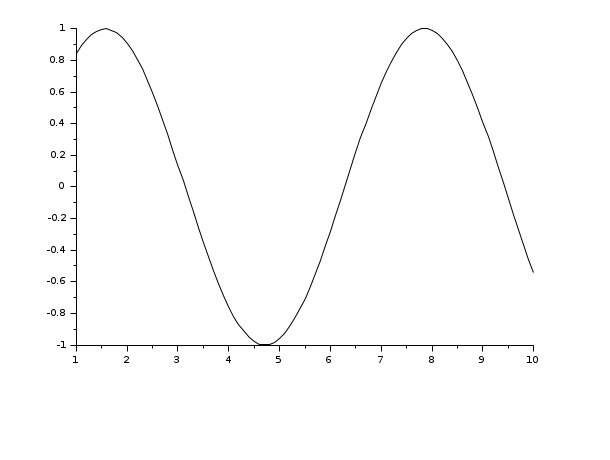# xsetech

set the sub-window of a graphics window for plotting

### Syntax

```xsetech(wrect, [frect, logflag])
xsetech(wrect=[...], frect=[..], logflag="..", arect=[...])
xsetech()```

### Arguments

wrect

a vector of size 4, defining the sub-window to use.

frect

a vector of size 4.

logflag

a string of size 2 `"xy"`, where `x` and `y` can be `"n"` or `"l"`. `"n"` stands for normal and "l" stands for logscale. `x` stands for the x-axis and `y` stands for the y-axis.

arect

a vector of size 4.

### Description

`xsetech` is mainly used to set the sub-window of the graphics window which will be used for plotting. The sub-window is specified with the parameter `wrect=[x,y,w,h]` (upper-left point, width, height). The values in `wrect` are specified using proportion of the width or height of the current graphic window. For instance `wrect=[0, 0, 1, 1]` means that the whole graphics window will be used, and `wrect=[0.5, 0, 0.5, 1]` means that the graphics region will be the right half of the graphics window.

`xsetech` also set the current graphics scales for 2D plotting and can be used in conjunction with graphics routines which request the current graphics scale (for instance `strf="x0z"` or `frameflag=0` in `plot2d`).

`frect=[xmin, ymin, xmax, ymax]` is used to set the graphics scale and is just like the `rect` argument of `plot2d`. If `frect` is not given the current value of the graphic scale remains unchanged. the default value of `rect` is `[0,0,1,1]` (at window creation, when switching back to default value with `clf('reset')` or when clearing graphic recorded events `clf()`).

`arect=[x_left, x_right,y_up,y_down]` is used to set the graphic frame inside the sub-window. The graphic frame is specified (like `wrect`) using proportion of the width or height of the current graphic sub-window. Default value is `1/8*[1,1,1,1]`. If `arect` is not given, current value remains unchanged.

### Examples

```// To get a graphical explanation of xsetech parameters enter:
exec('SCI/modules/graphics/demos/xsetechfig.sce');

// Here xsetech is used to split the graphics window in two parts
// first xsetech is used to set the first sub-window
// and the graphics scale
xsetech([0,0,1.0,0.5],[-5,-3,5,3])
// we call plot2d with the "001" option to use the graphics scale
// set by xsetech
plot2d([1:10]',[1:10]',1,"001"," ")
// then xsetech is used to set the second sub-window
xsetech([0,0.5,1.0,0.5])
// the graphics scale is set by xsetech to [0,0,1,1] by default
// and we change it with the use of the rect argument in plot2d
plot2d([1:10]',[1:10]',1,"011"," ",[-6,-6,6,6])```
```// Four plots on a single graphics window
clf()
set(gca(),"font_style",2,"font_size",0);
xsetech([0,0,0.5,0.5]);
plot3d()
xsetech([0.5,0,0.5,0.5]);
plot2d()
xsetech([0.5,0.5,0.5,0.5]);
grayplot()
xsetech([0,0.5,0.5,0.5]);
histplot()
// back to default values for the sub-window
xsetech([0,0,1,1])``````// One plot with changed arect
clf()
xsetech(arect=[0,0,0,0])
x=1:0.1:10;plot2d(x',sin(x)')``````clf()
xsetech(arect=[1/8,1/8,1/16,1/4])
x=1:0.1:10;plot2d(x',sin(x)')
clf()```